The major difference between lattice and ggplot2 is that lattice uses a formula based interface. ggplot2 does not because the formula does not generalise well to more complicated situations.

## Examples

library(lattice)

if (require("ggplot2movies")) {
xyplot(rating ~ year, data=movies)
qplot(year, rating, data=movies)

xyplot(rating ~ year | Comedy + Action, data = movies)
qplot(year, rating, data = movies, facets = ~ Comedy + Action)
# Or maybe
qplot(year, rating, data = movies, facets = Comedy ~ Action)

# While lattice has many different functions to produce different types of
# graphics (which are all basically equivalent to setting the panel argument),
# ggplot2 has qplot().

stripplot(~ rating, data = movies, jitter.data = TRUE)
qplot(rating, 1, data = movies, geom = "jitter")

histogram(~ rating, data = movies)
qplot(rating, data = movies, geom = "histogram")

bwplot(Comedy ~ rating ,data = movies)
qplot(factor(Comedy), rating, data = movies, geom = "boxplot")

xyplot(wt ~ mpg, mtcars, type = c("p","smooth"))
qplot(mpg, wt, data = mtcars, geom = c("point","smooth"))
}#> geom_smooth() using method = 'loess' and formula 'y ~ x'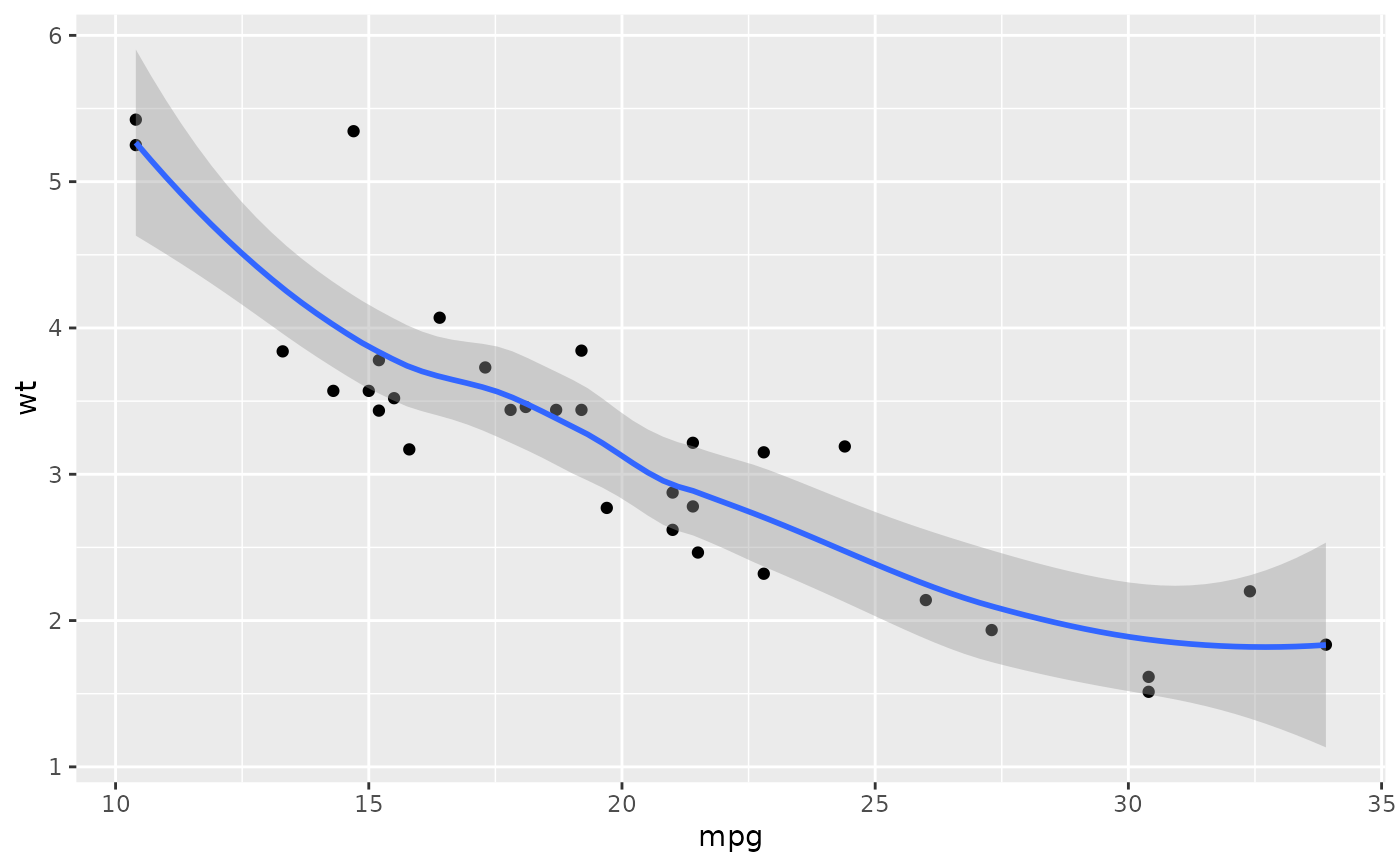# The capabilities for scale manipulations are similar in both ggplot2 and
# lattice, although the syntax is a little different.

xyplot(wt ~ mpg | cyl, mtcars, scales = list(y = list(relation = "free")))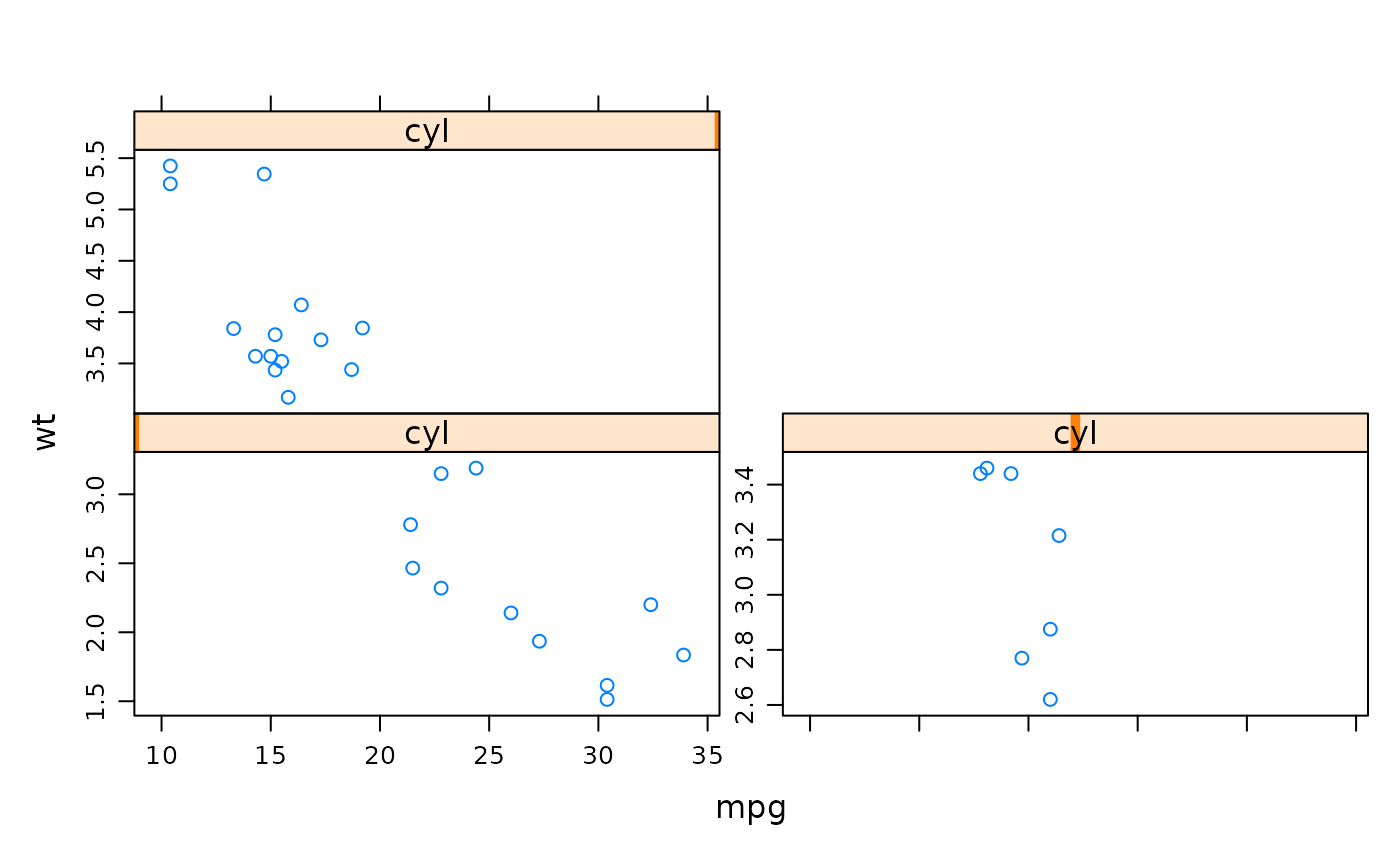qplot(mpg, wt, data = mtcars) + facet_wrap(~ cyl, scales = "free")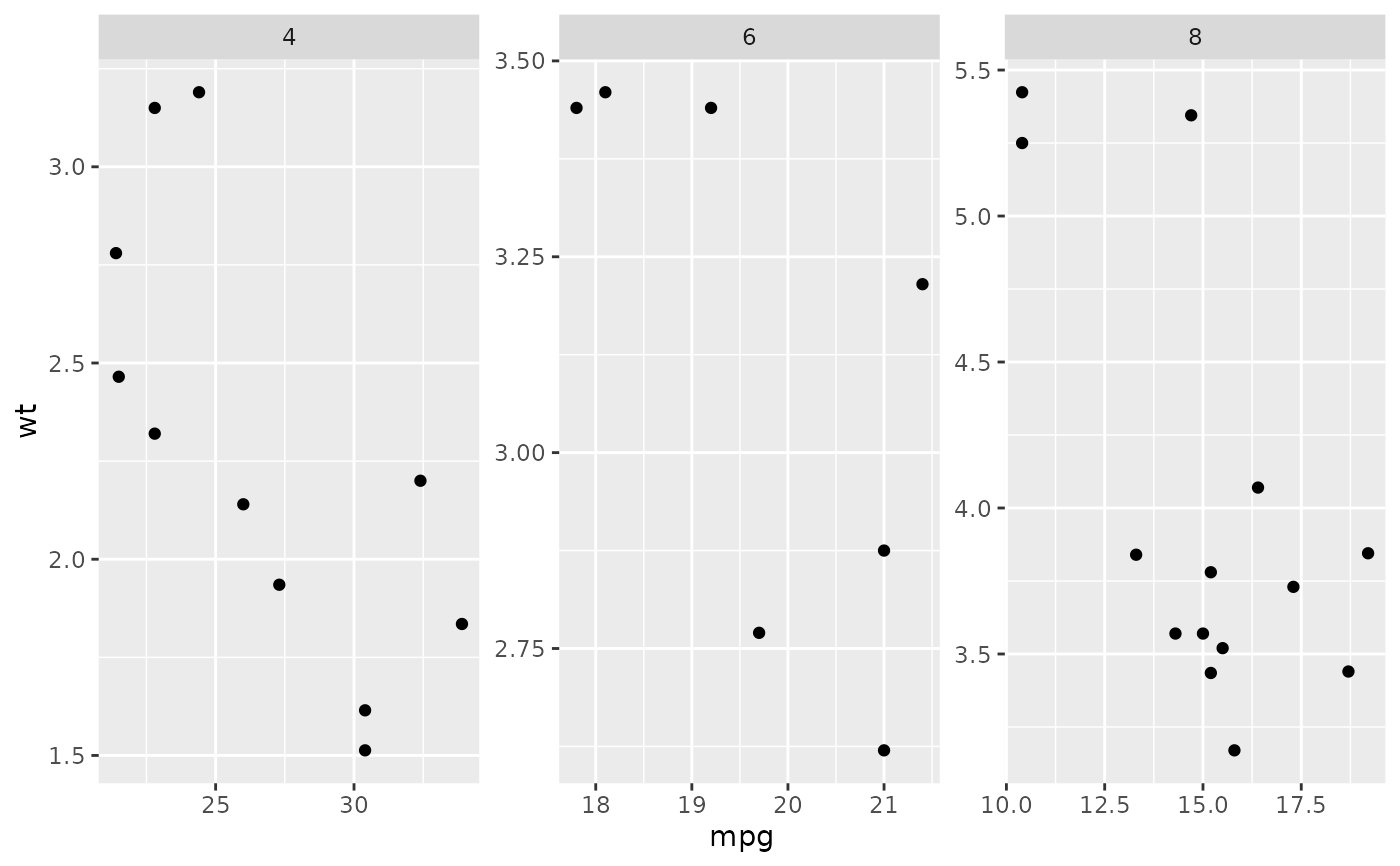xyplot(wt ~ mpg | cyl, mtcars, scales = list(log = 10))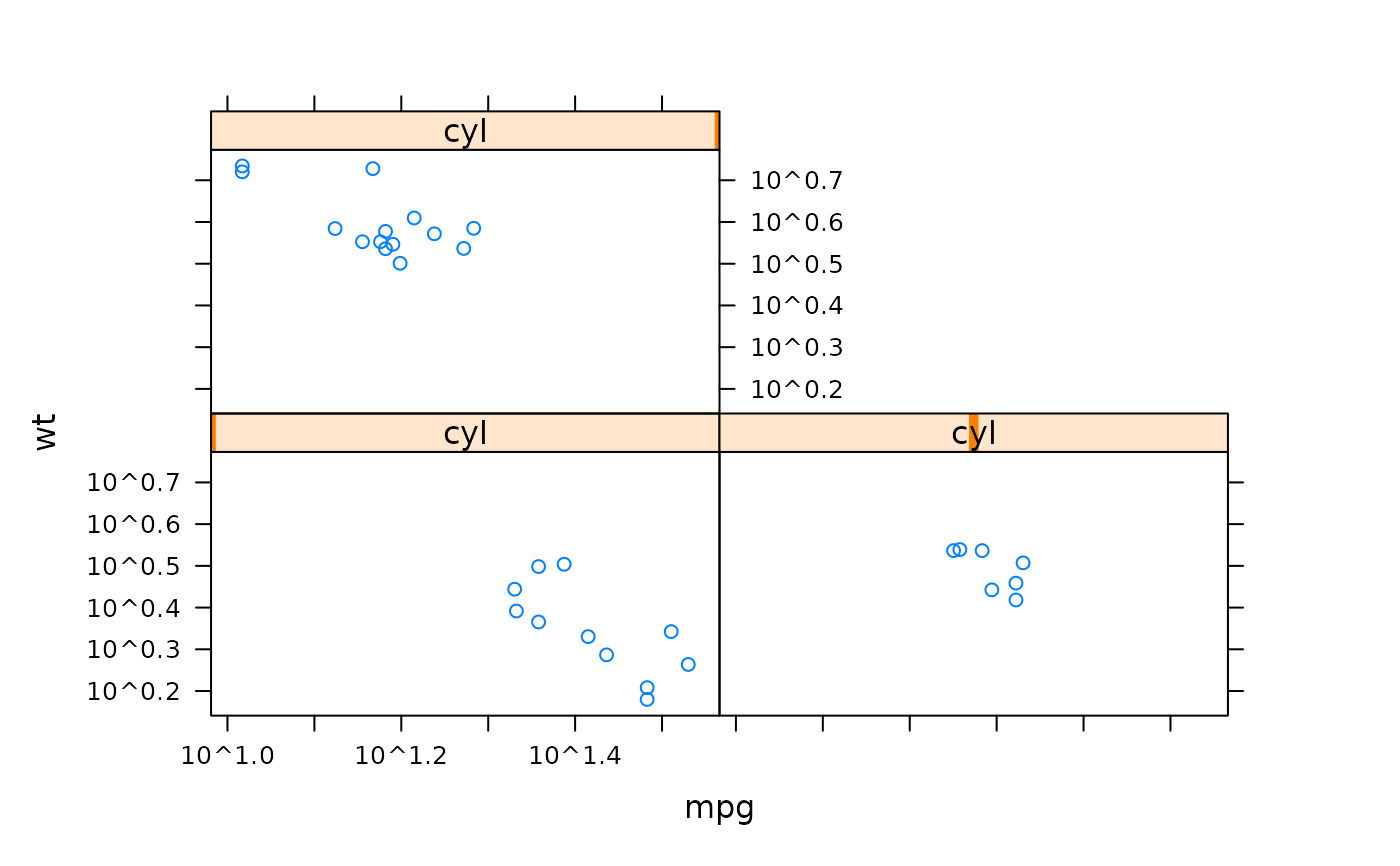qplot(mpg, wt, data = mtcars, log = "xy")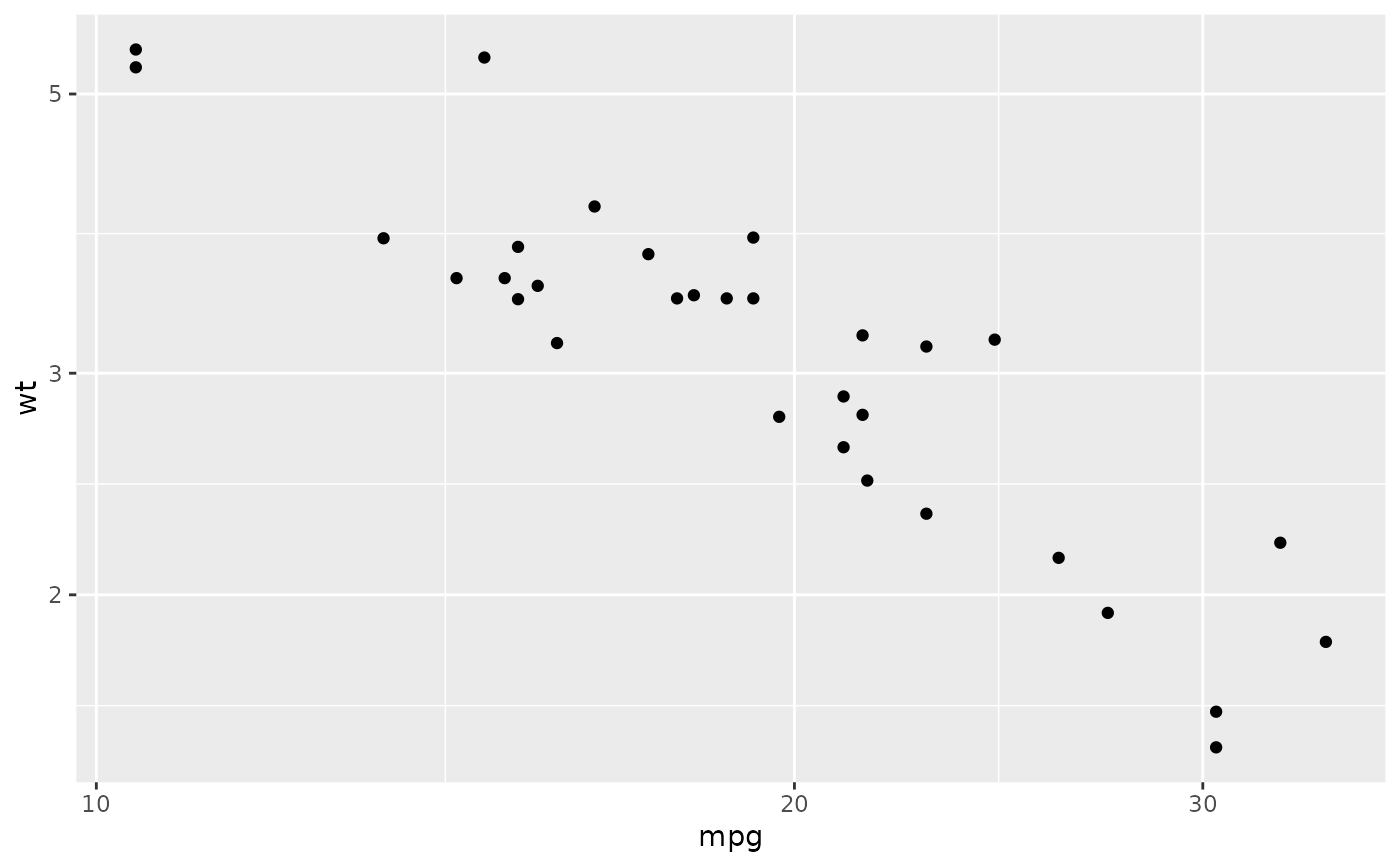xyplot(wt ~ mpg | cyl, mtcars, scales = list(log = 2))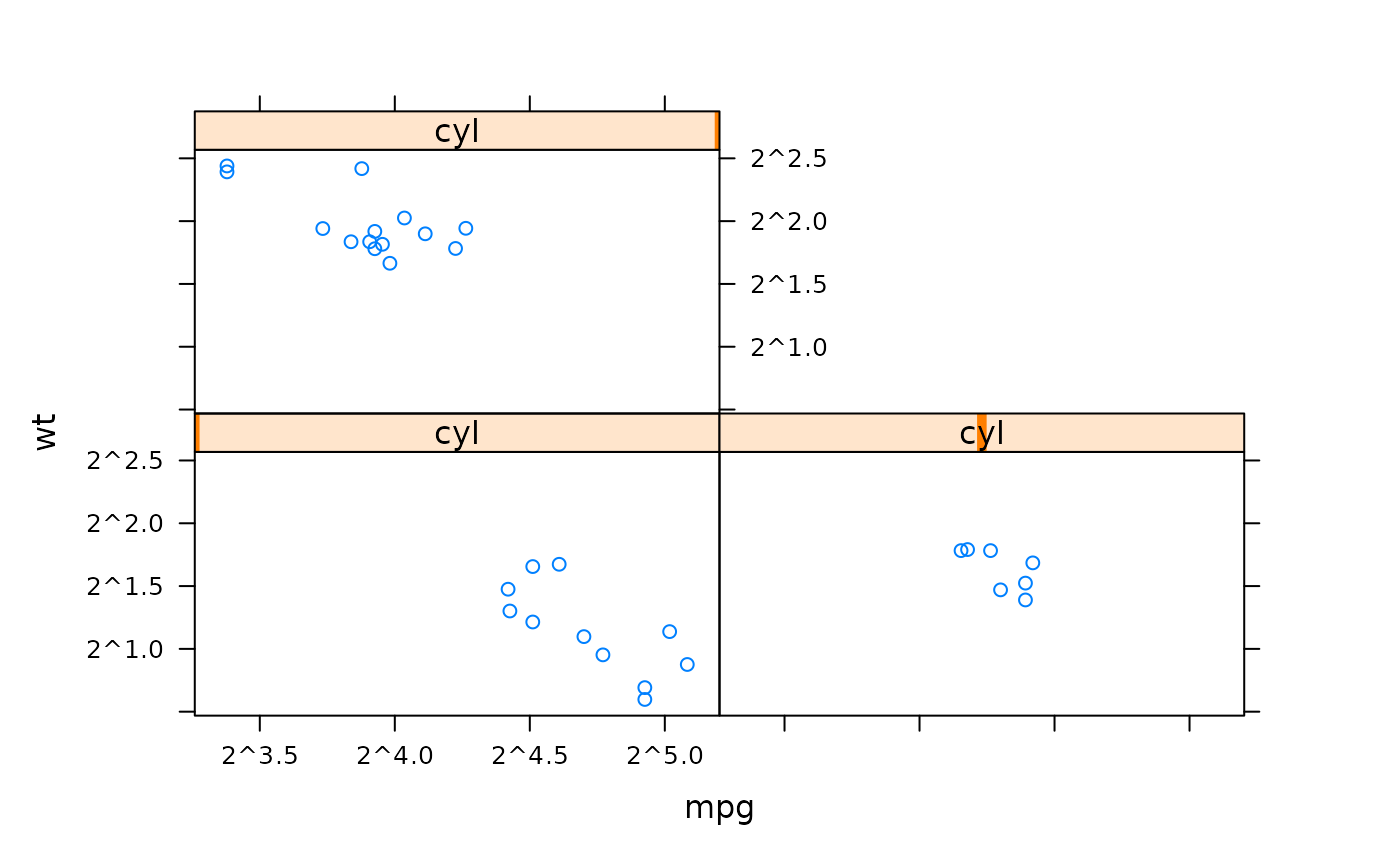qplot(mpg, wt, data = mtcars) +
scale_x_continuous(trans = scales::log2_trans()) +
scale_y_continuous(trans = scales::log2_trans())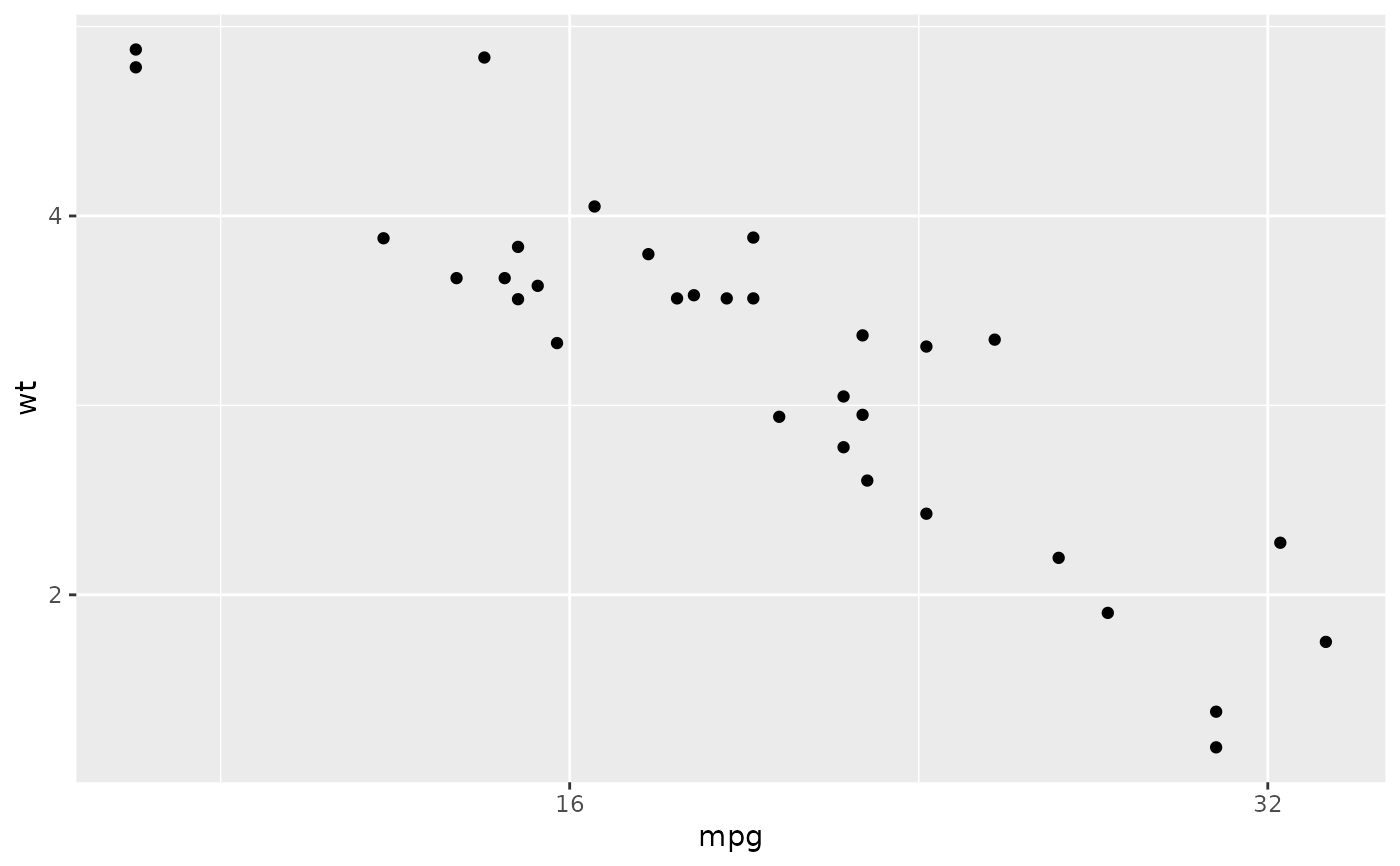xyplot(wt ~ mpg, mtcars, group = cyl, auto.key = TRUE)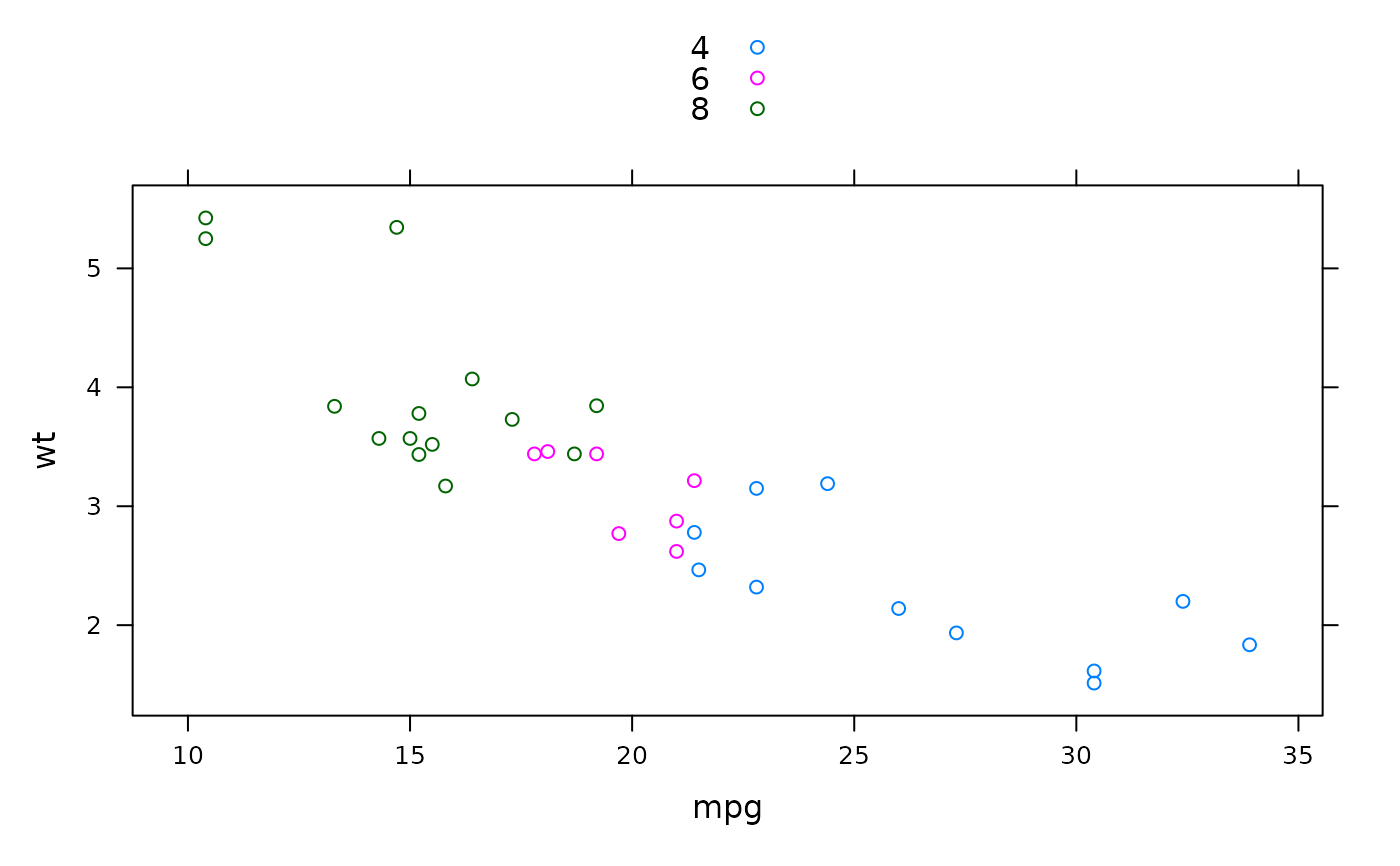# Map directly to an aesthetic like colour, size, or shape.
qplot(mpg, wt, data = mtcars, colour = cyl)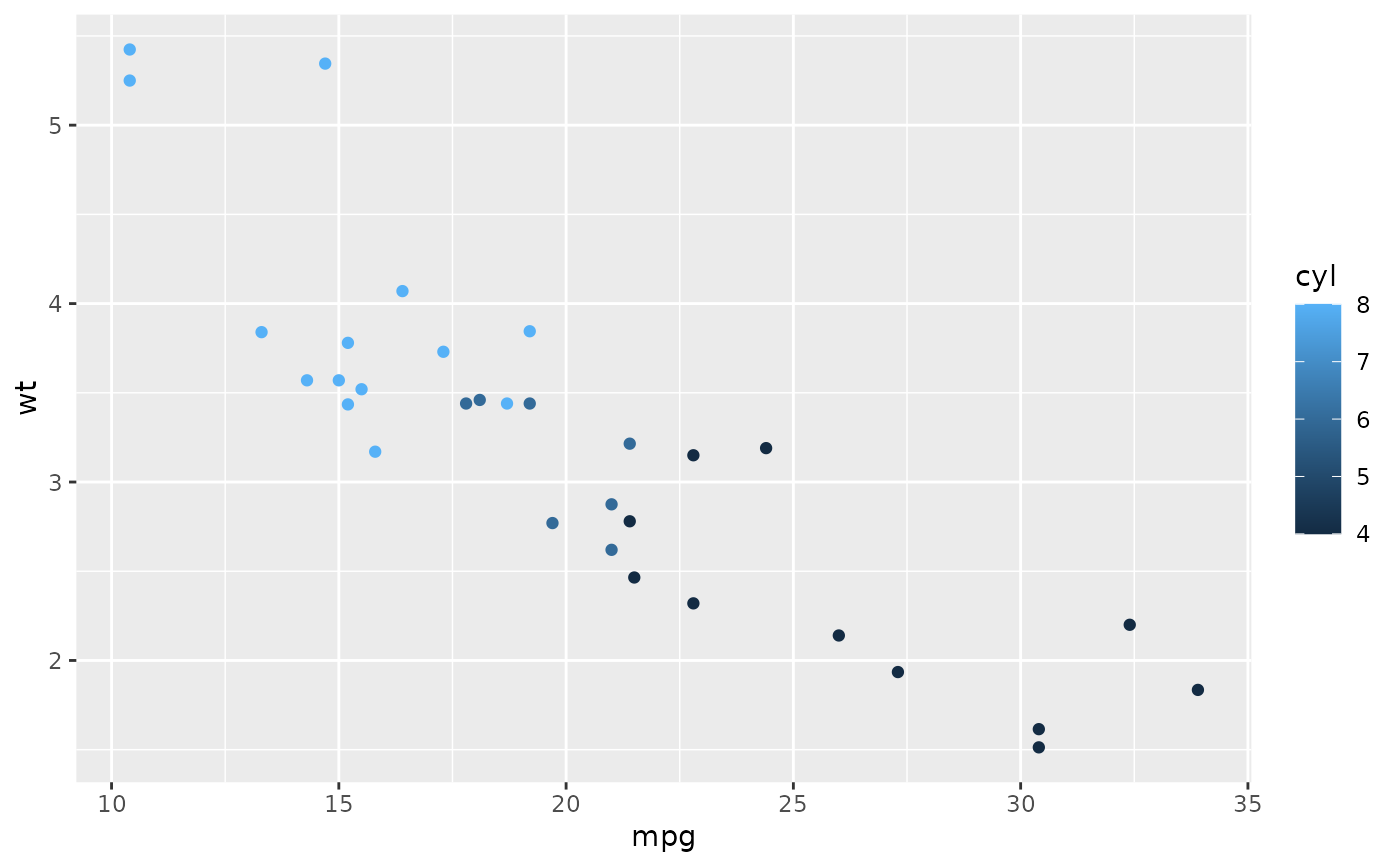xyplot(wt ~ mpg, mtcars, xlim = c(20,30))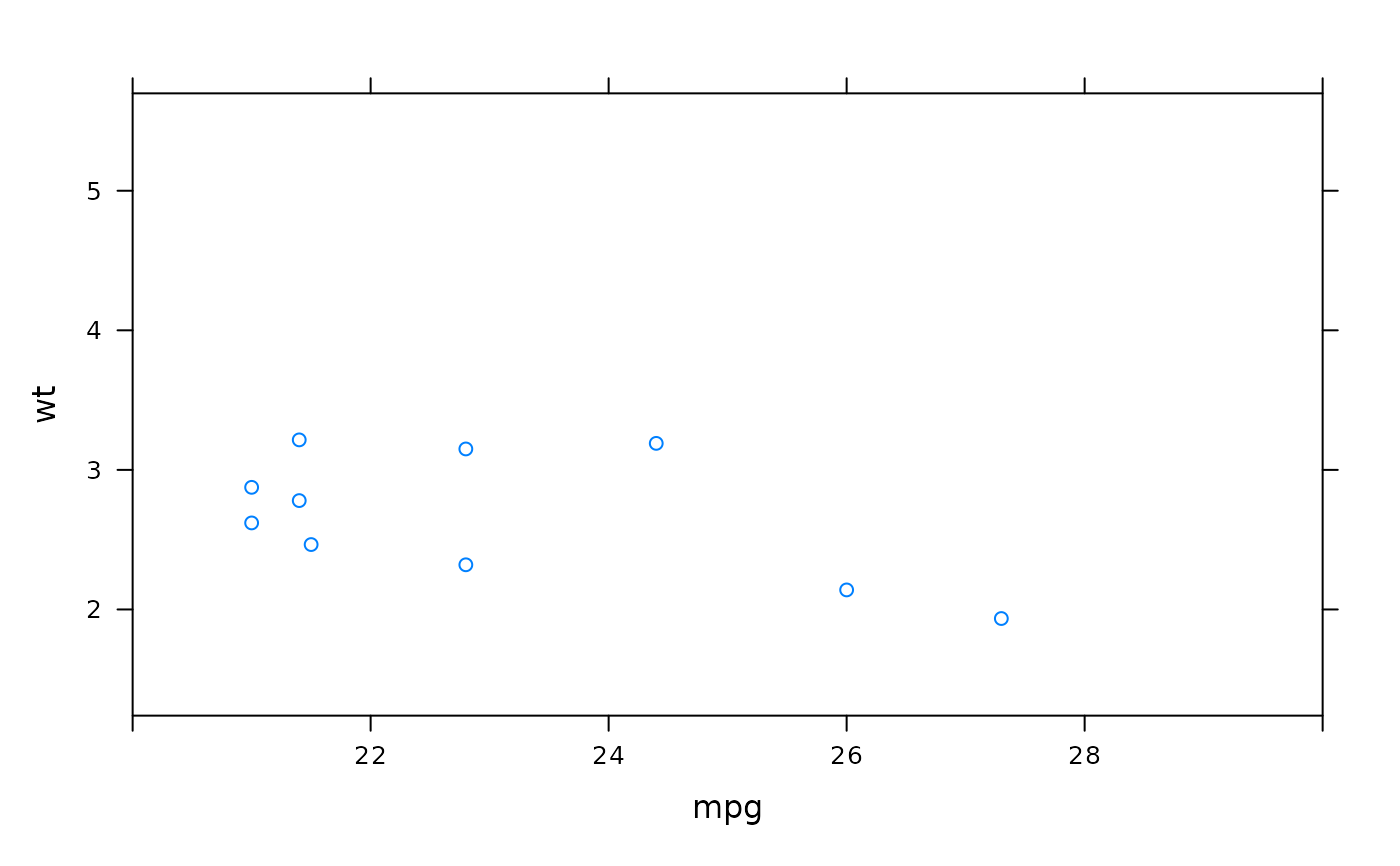# Works like lattice, except you can't specify a different limit
# for each panel/facet
qplot(mpg, wt, data = mtcars, xlim = c(20,30))#> Warning: Removed 22 rows containing missing values (geom_point).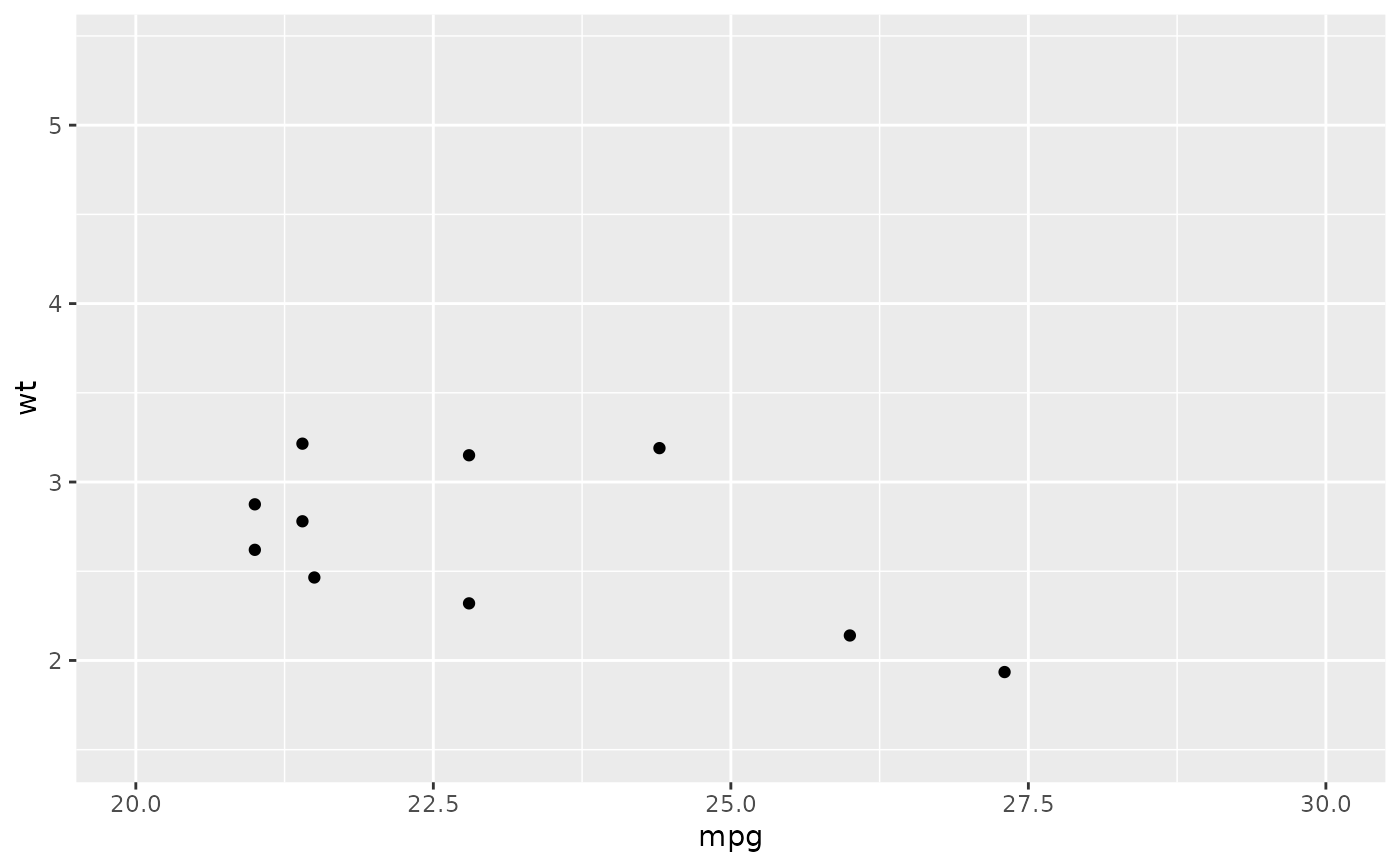# Both lattice and ggplot2 have similar options for controlling labels on the plot.

xyplot(wt ~ mpg, mtcars, xlab = "Miles per gallon", ylab = "Weight",
main = "Weight-efficiency tradeoff")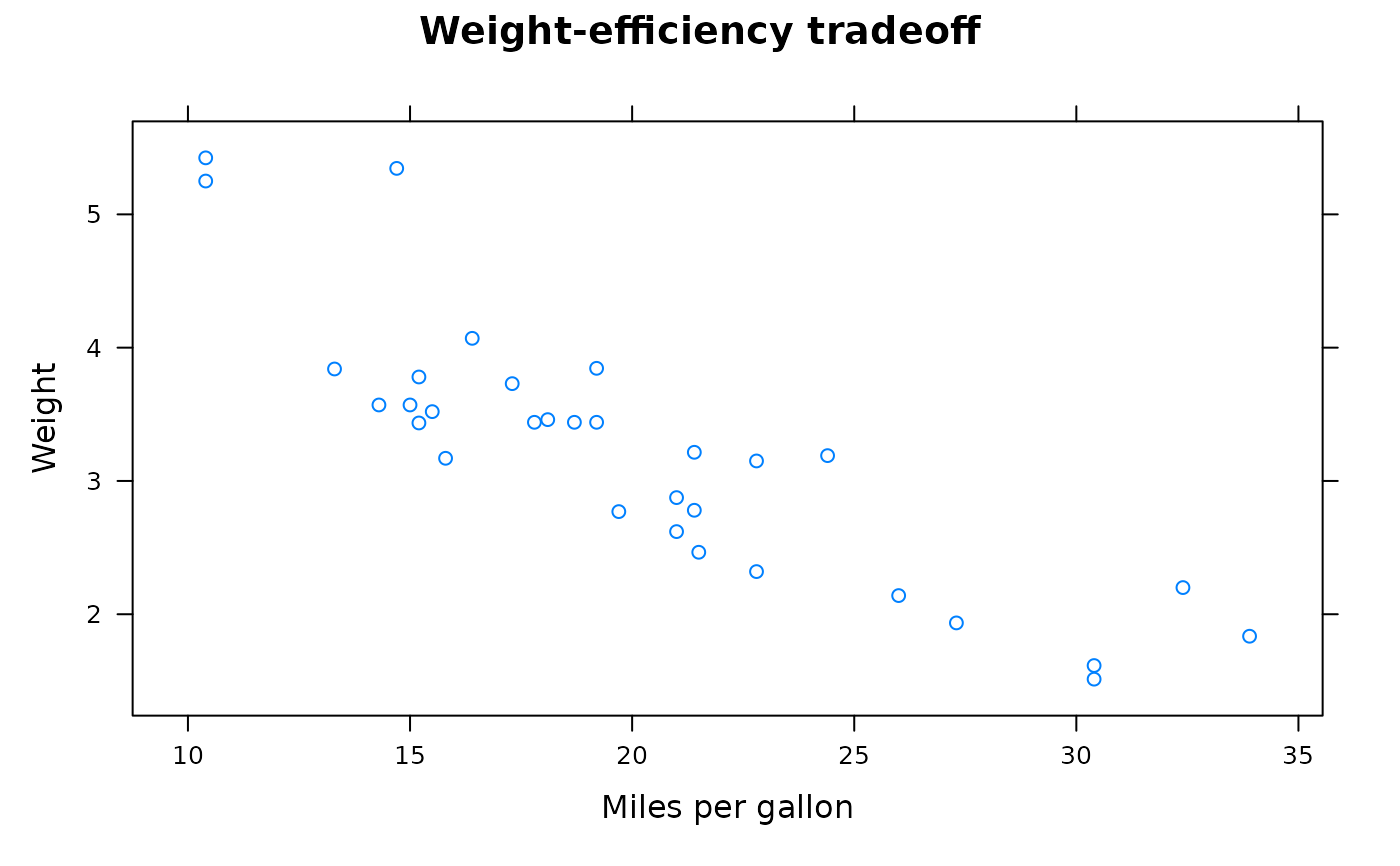qplot(mpg, wt, data = mtcars, xlab = "Miles per gallon", ylab = "Weight",
main = "Weight-efficiency tradeoff")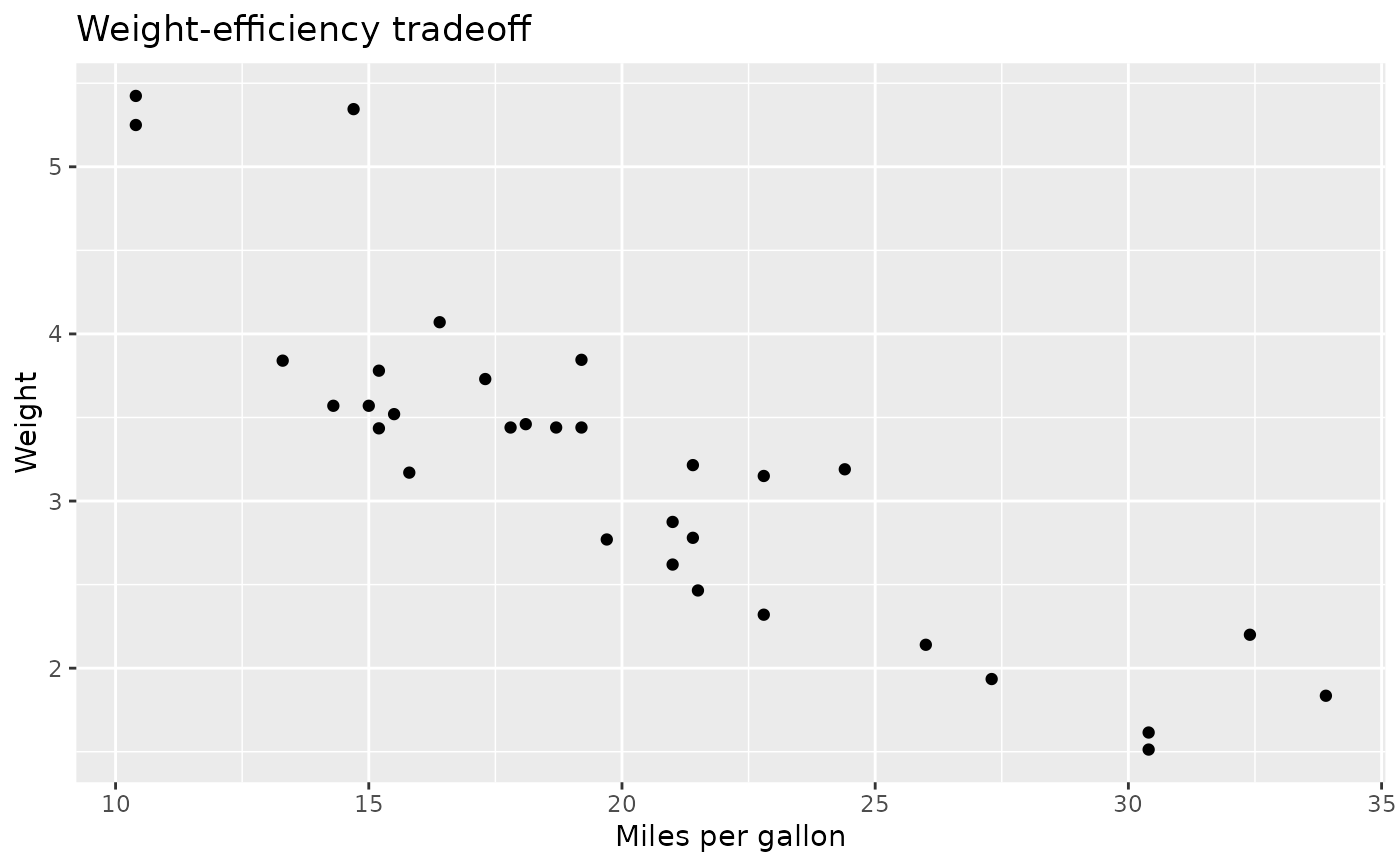xyplot(wt ~ mpg, mtcars, aspect = 1)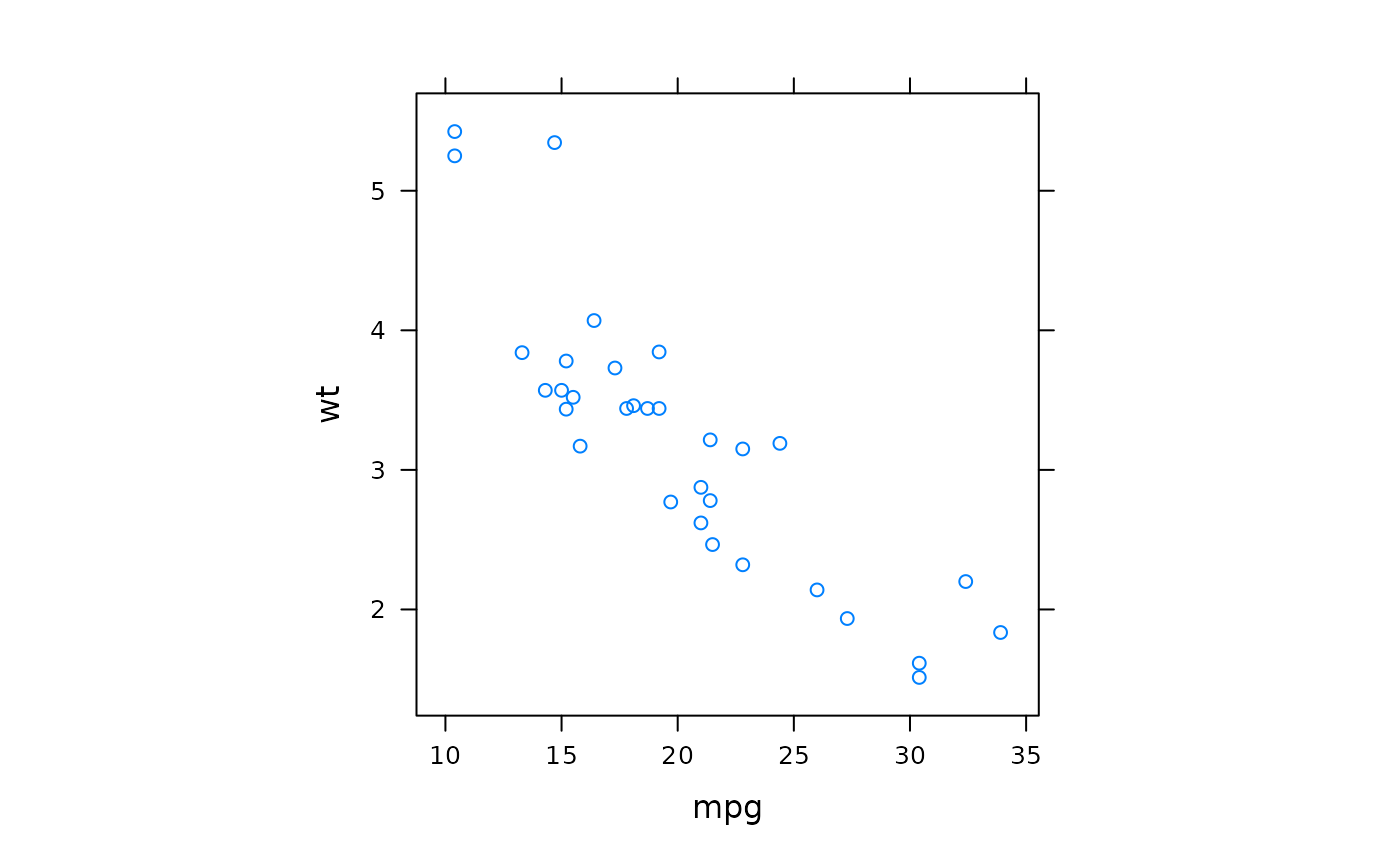qplot(mpg, wt, data = mtcars, asp = 1)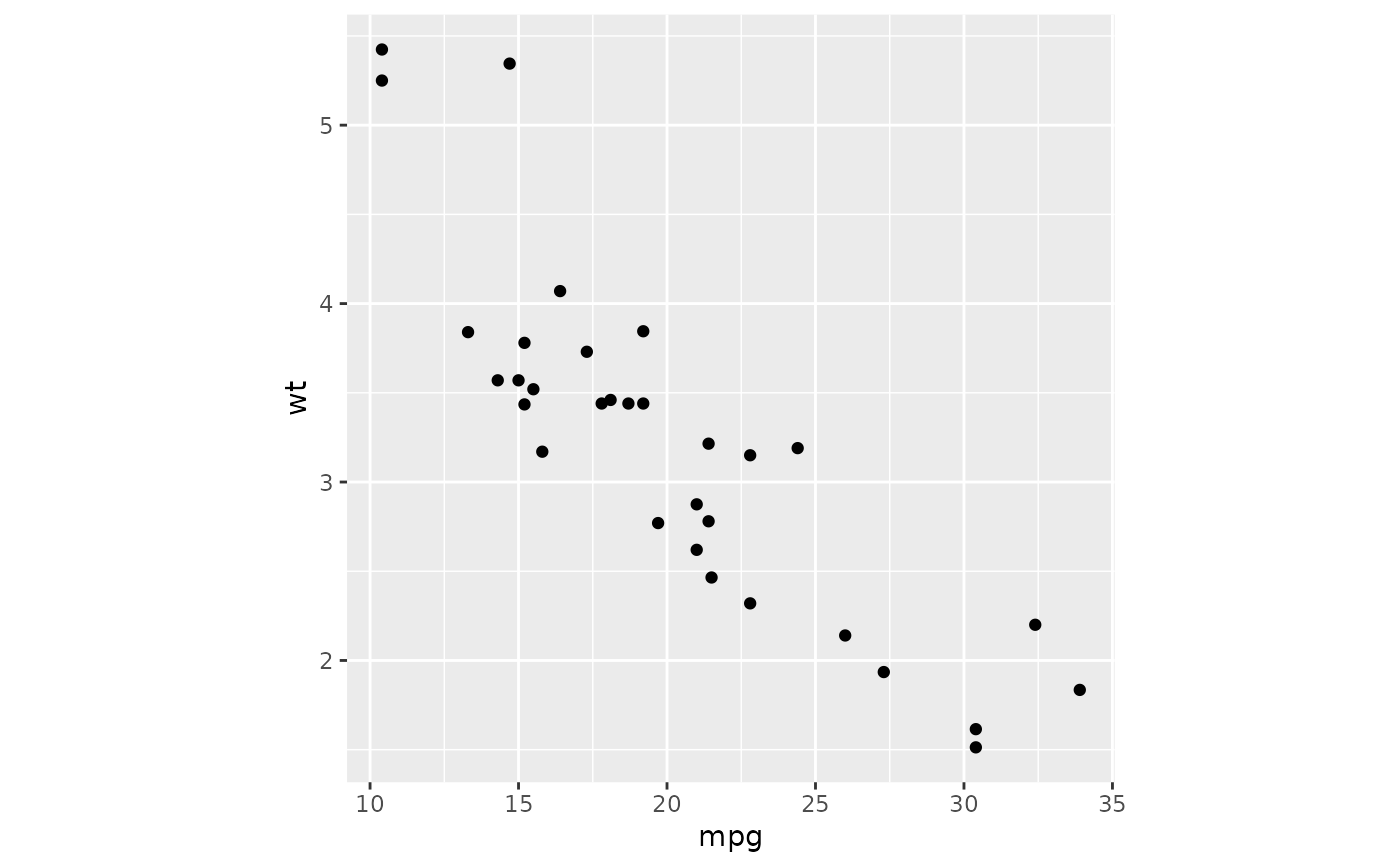# par.settings() is equivalent to + theme() and trellis.options.set()
# and trellis.par.get() to theme_set() and theme_get().
# More complicated lattice formulas are equivalent to rearranging the data
# before using ggplot2.